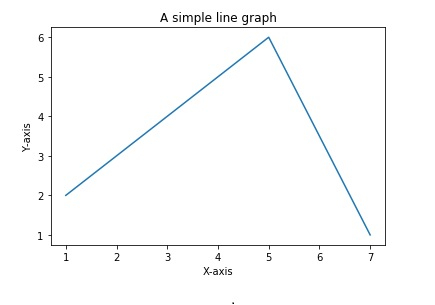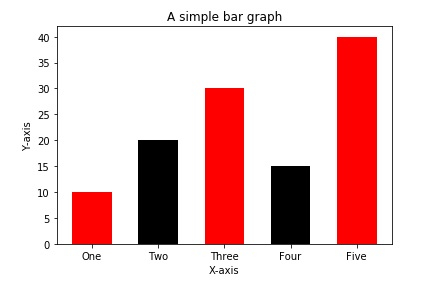# How to plot a graph in Python?

Graphs in Python can be plotted by using the Matplotlib library. Matplotlib library is mainly used for graph plotting.

You need to install matplotlib before using it to plot graphs. Matplotlib is used to draw a simple line, bargraphs, histograms and piecharts. Inbuilt functions are available to draw all types of graphs in the matplotlib library.

## Plot a line in a graph

We will plot a simple line in a graph using matplotlib. The following steps are involved in plotting a line.

• Import matplotlib

• Specify the x-coordinates and y-coordinates of the line

• Plot the specified points using specific function using .plot() function

• Name the x-axis and y-axis using .xlabel() and .ylabel() functions

• Give a title to the graph(optional) using .title() function

• Show the graph using .show() function

These are the simple steps involved in plotting a line using matplotlib.

### Example

import matplotlib.pyplot as plt

x=[1,3,5,7]
y=[2,4,6,1]
plt.plot(x,y)
plt.xlabel('X-axis')
plt.ylabel('Y-axis')
plt.title("A simple line graph")
plt.show()

The above code plots the points (1,2),(3,4),(5,6),(7,1) and joins these points with a line which is shown as the graph.

### Output## Plot a bar graph

A bar graph is the way of representing data by rectangles of different heights at specific positions on the x-axis.

The following steps are involved in drawing a bar graph −

• Import matplotlib

• Specify the x-coordinates where the left bottom corner of the rectangle lies.

• Specify the heights of the bars or rectangles.

• Specify the labels for the bars

• Plot the bar graph using .bar() function

• Give labels to the x-axis and y-axis

• Give a title to the graph

• Show the graph using .show() function.

### Example

import matplotlib.pyplot as plt

left_coordinates=[1,2,3,4,5]
heights=[10,20,30,15,40]
bar_labels=['One','Two','Three','Four','Five']
plt.bar(left_coordinates,heights,tick_label=bar_labels,width=0.6,color=['re
d','black'])
plt.xlabel('X-axis')
plt.ylabel('Y-axis')
plt.title("A simple bar graph")
plt.show()

The width parameter in the plt.bar() specifies the width of each bar. The color lists specify the colors of the bars.

### Output